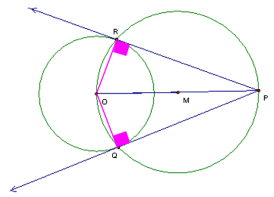Tangents to a Circle

Step-by-step directions for constructing tangents to a circle from a point
not on the circle using Geometer's Sketchpad.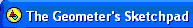Version 4.+
Construct a tangent to a circle from a point not on the circle.

1.  Use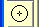to draw a circle.  Label it O.  Right clicking on a point will allow fast labeling if automatic labeling it not turned on.  To turn on Automatic Labeling, choose Edit, Preferences, Text Tab, check the box under Show Labels Automatically "For All New Points".

2.  Useto locate a point not on the circle.  Label it P.

3.  Selectpoints O and P by clicking on them.  Use the segment command under the construct menu to connect O to P.

4.  Select the segment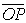by clicking on it.  Use the "Midpoint" command under the "Construct" menu to bisect the segment.  Label the point M.

5.  Select points O and M.  Use the "Segment" command under the "Construct" menu to create a segment from O to M.

6.  Select segment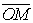and point M.  Use the "Circle By Center+Radius" under the "Construct" menu to construct circle M with radius.  Label the intersections with circle O as points R and Q.

7.  Select P and then R.  Use the "Ray" command under the "Construct" menu to draw a ray.  Select Q and then R.  Repeat drawing a ray.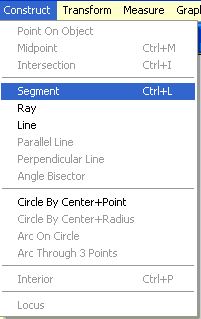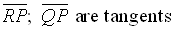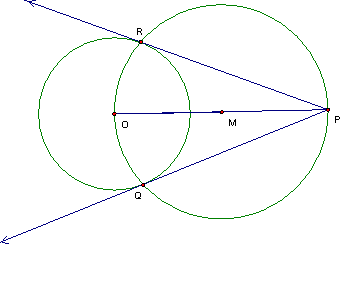Does this really work?  Are these really tangents?

 Answer:  YES Draw a segment from O to R and from O to Q forming radii in circle O.  Angles ∠ORP and ∠OQP are right angles because an angle inscribed in a semi-circle (in circle M) is a right angle.  The presence of these right angles means that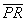is perpendicular to radius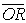in circle O.  The same is true for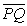being perpendicular to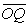in circle O.   Sinceandare perpendicular to radiiandat the point of contact on circle O, segmentsand 19 are tangents to circle O.